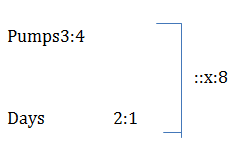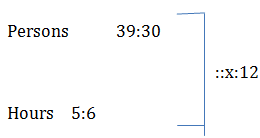# 3. Chain Rule; Quantitative Aptitude; Civil Service Examination; RPSC Notes | Study RAS RPSC Prelims Preparation - Notes, Study Material & Tests - UPSC

## UPSC: 3. Chain Rule; Quantitative Aptitude; Civil Service Examination; RPSC Notes | Study RAS RPSC Prelims Preparation - Notes, Study Material & Tests - UPSC

The document 3. Chain Rule; Quantitative Aptitude; Civil Service Examination; RPSC Notes | Study RAS RPSC Prelims Preparation - Notes, Study Material & Tests - UPSC is a part of the UPSC Course RAS RPSC Prelims Preparation - Notes, Study Material & Tests.
All you need of UPSC at this link: UPSC

This module will teach you the basics of direct and indirect proportions. These concepts will further help you in time and work questions.

Important Formulas – chain rule

• Direct Proportion

Two quantities are said to be directly proportional, if on the increase or decrease of the one, the other increases or decreases the same extent.

Examples

1. Cost of the goods is directly proportional to the number of goods. (More goods, More cost)
2. Amount of work done is directly proportional to the number of persons who did the work. (More persons, More Work)
• Indirect Proportion (inverse proportion)

Two quantities are said to be indirectly proportional (inversely proportional) if on the increase of the one, the other decreases to the same extent and vice-versa.

Examples

• Number of days needed to complete a work is indirectly proportional (inversely proportional) with the number of persons who does the work (More Persons, Less Days needed)
• The time taken to travel a distance is indirectly proportional (inversely proportional) with the speed in which one is travelling (More Speed, Less Time)

Solved Examples

 1. If the cost of x metres of wire is d rupees, then what is the cost of y metres of wire at the same rate? A. Rs. (xd/y) B. Rs. x/d C. Rs. (yd/x) D. Rs. y/d

Explanation :

cost of x metres of wire = Rs. d

cost of 1 metre of wire = Rs.(d/x)

cost of y metre of wire = Rs.(y×d/x)=Rs. (yd/x)

 2. In a camp, there is a meal for 120 men or 200 children. If 150 children have taken the meal, how many men will be catered to with remaining meal? A. 50 B. 30 C. 40 D. 10

Explanation :

Meal for 200 children = Meal for 120 men

Meal for 1 child = Meal for 120/200 men

Meal for 150 children = Meal for (120×150)/200 men=Meal for 90 men

Total mean available = Meal for 120 men

Renaming meal = Meal for 120 men - Meal for 90 men = Meal for 30 men

 3. 36 men can complete a piece of work in 18 days. In how many days will 27 men complete the same work? A. 26 B. 22 C. 12 D. 24

Explanation :

Let the required number of days be x

More men, less days (indirect proportion)

Hence we can write as

Men36:27}::x:18 ⇒36×18=27×x ⇒12×18=9×x

⇒12×2=x

⇒x=24

 4. A wheel that has 6 cogs is meshed with a larger wheel of 14 cogs. If the smaller wheel has made 21 revolutions, what will be the number of revolutions made by the larger wheel? A. 15 B. 12 C. 21 D. 9

Explanation :

Let the number of revolutions made by the larger wheel be x

More cogs, less revolutions (Indirect proportion)

Hence we can write as

Cogs 6:14}: x: 21 ⇒6×21=14×x ⇒6×3=2×x ⇒3×3=x ⇒x=9

 5. 3 pumps, working 8 hours a day, can empty a tank in 2 days. How many hours a day should 4 pumps work in order to empty the tank in 1 day? A. 10 B. 12 C. 8 D. 15

Explanation :

Let the required hours needed be x

More pumps, less hours (Indirect proportion)
More Days, less hours (Indirect proportion)

Hence we can write as⇒3×2×8=4×1×x

⇒3×2×2=x

⇒x=12

 6. 39 persons can repair a road in 12 days, working 5 hours a day. In how many days will 30 persons, working 6 hours a day, complete the work? A. 9 B. 12 C. 10 D. 13

Explanation :
Let the required number of days be x

More hours, less days (indirect proportion)

Hence we can write as⇒39×5×12=30×6×x ⇒39×5×2=30×x ⇒39=3×x ⇒x=13

 7. A certain industrial loom weaves 0.128 meters of cloth every second. Approximately how many seconds will it take for the loom to weave 25 meters of cloth? A. 205 B. 200 C. 180 D. 195

Explanation :

Let the required number of seconds be x

More cloth, More time, (direct proportion)

Hence we can write as

Cloth         0.128:25} :: 1:x

⇒0.128x=25 ⇒x=25/0.128 ⇒25000/128=3125/16≈195

 8. 21 goats eat as much as 15 cows. How many goats each as much as 35 cows? A. 49 B. 32 C. 36 D. 41

Explanation :

15 cows ≡ 21 goats

1 cow ≡21/15 goats

35 cows ≡ (21×35)/15 goats≡(21×7)/3 goats≡7×7 goats ≡ 49 goats

The document 3. Chain Rule; Quantitative Aptitude; Civil Service Examination; RPSC Notes | Study RAS RPSC Prelims Preparation - Notes, Study Material & Tests - UPSC is a part of the UPSC Course RAS RPSC Prelims Preparation - Notes, Study Material & Tests.
All you need of UPSC at this link: UPSCUse Code STAYHOME200 and get INR 200 additional OFF

## RAS RPSC Prelims Preparation - Notes, Study Material & Tests

109 docs|21 tests

### How to Prepare for UPSC

Read our guide to prepare for UPSC which is created by Toppers & the best Teachers

Track your progress, build streaks, highlight & save important lessons and more!

,

,

,

,

,

,

,

,

,

,

,

,

,

,

,

,

,

,

,

,

,

,

,

,

;